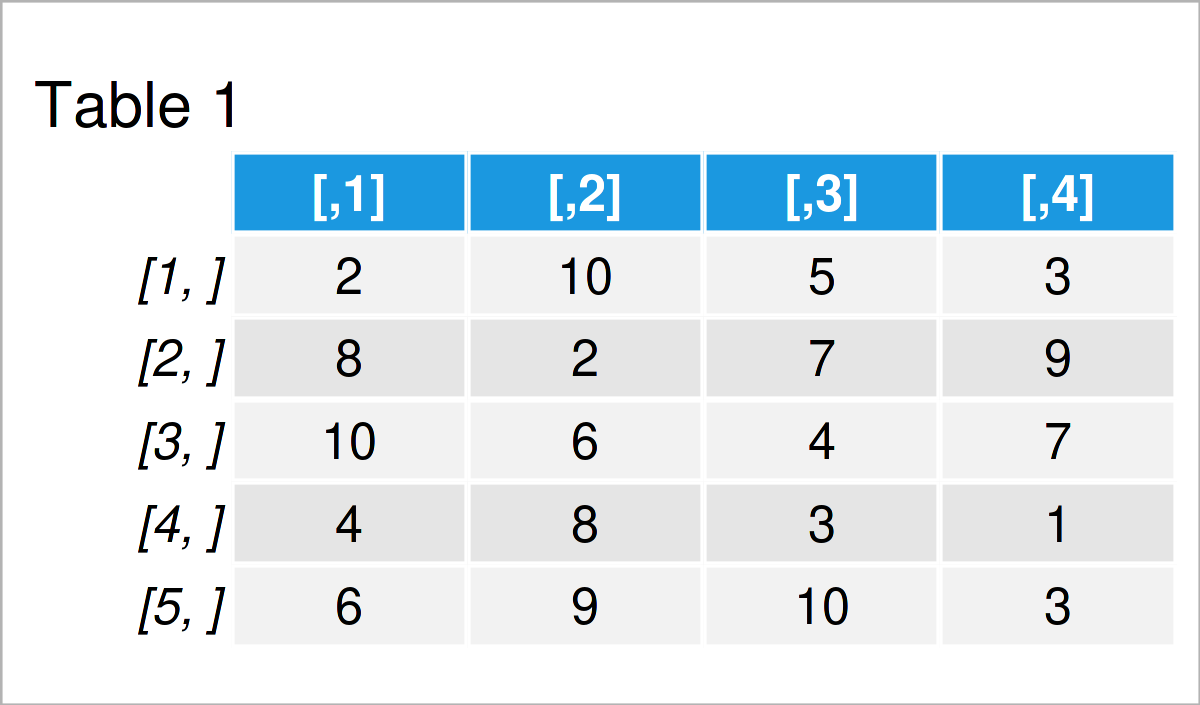# How to Calculate the Norm of a Matrix in R (5 Examples) | norm() Function

In this R programming tutorial you’ll learn how to compute the norm of a matrix using the norm() function.

The content of the tutorial looks like this:

Let’s dig in:

## Creation of Example Data

We’ll use the following data as basement for this R tutorial:

```set.seed(3826733)               # Create example matrix
my_mat <- matrix(sample(1:10, replace = TRUE, 20), ncol = 4)
my_mat                          # Print example matrix```Have a look at the previous table. It shows that our example data contains five rows and four integer columns.

Let’s compute different types of the norm of this matrix.

## Example 1: Compute One Norm of Matrix

Example 1 explains how to calculate the one norm of a matrix, i.e. the default specification of the norm function.

Have a look at the following R code:

```norm(my_mat)                    # One norm
#  35```

The RStudio console has returned the one norm of our example matrix, i.e. 35.

## Example 2: Compute Infinity Norm of Matrix

The norm function provides different types of matrix norms.

Example 2 shows how to get the infinity norm of a matrix:

```norm(my_mat, type = "I")        # Infinity norm
#  28```

## Example 3: Compute Forbenius Norm of Matrix

This example illustrates how to return the Forbenius norm of a matrix:

```norm(my_mat, type = "F")        # Forbenius norm
#  29.20616```

## Example 4: Compute Maximum Modulus Norm of Matrix

This example explains how to compute the maximum modulus norm of a matrix:

```norm(my_mat, type = "M")        # Maximum modulus
#  10```

## Example 5: Compute Spectral Norm / 2-Norm of Matrix

This example demonstrates how to calculate the spectral norm (or 2-norm) of a data matrix.

```norm(my_mat, type = "2")        # Spectral / 2-norm
#  26.84743```

## Video & Further Resources

Do you need further information on the R programming codes of this tutorial? Then you may have a look at the following video on my YouTube channel. I’m explaining the content of this tutorial in the video.

In addition, you may have a look at the other posts on this homepage. I have published several articles already:

Summary: In this post you have learned how to apply the norm() function in R. Please let me know in the comments section below, if you have any further questions.

Subscribe to the Statistics Globe Newsletter